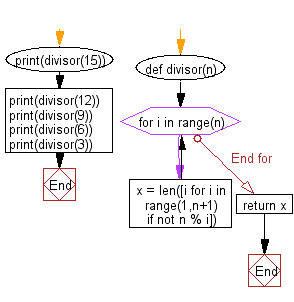﻿ Python: Find the number of divisors of a given integer is even or odd - w3resource# Python: Find the number of divisors of a given integer is even or odd

## Python Basic - 1: Exercise-24 with Solution

Write a Python program to find the number of divisors of a given integer is even or odd.

Sample Solution:

Python Code:

``````def divisor(n):
for i in range(n):
x = len([i for i in range(1,n+1) if not n % i])
return x
print(divisor(15))
print(divisor(12))
print(divisor(9))
print(divisor(6))
print(divisor(3))
``````

Sample Output:

```4
6
3
4
2
```

Pictorial Presentation:Flowchart:## Visualize Python code execution:

The following tool visualize what the computer is doing step-by-step as it executes the said program:

Python Code Editor:

Have another way to solve this solution? Contribute your code (and comments) through Disqus.

What is the difficulty level of this exercise?

Test your Python skills with w3resource's quiz

﻿

## Python: Tips of the Day

Checks if the given number falls within the given range.

Example:

```def tips_range(n, start, end = 0):
return start <= n <= end if end >= start else end <= n <= start
print(tips_range(2, 4, 6))
print(tips_range(4, 8))
print(tips_range(1, 3, 5))
print(tips_range(1, 3))
```

Output:

```False
True
False
True
```